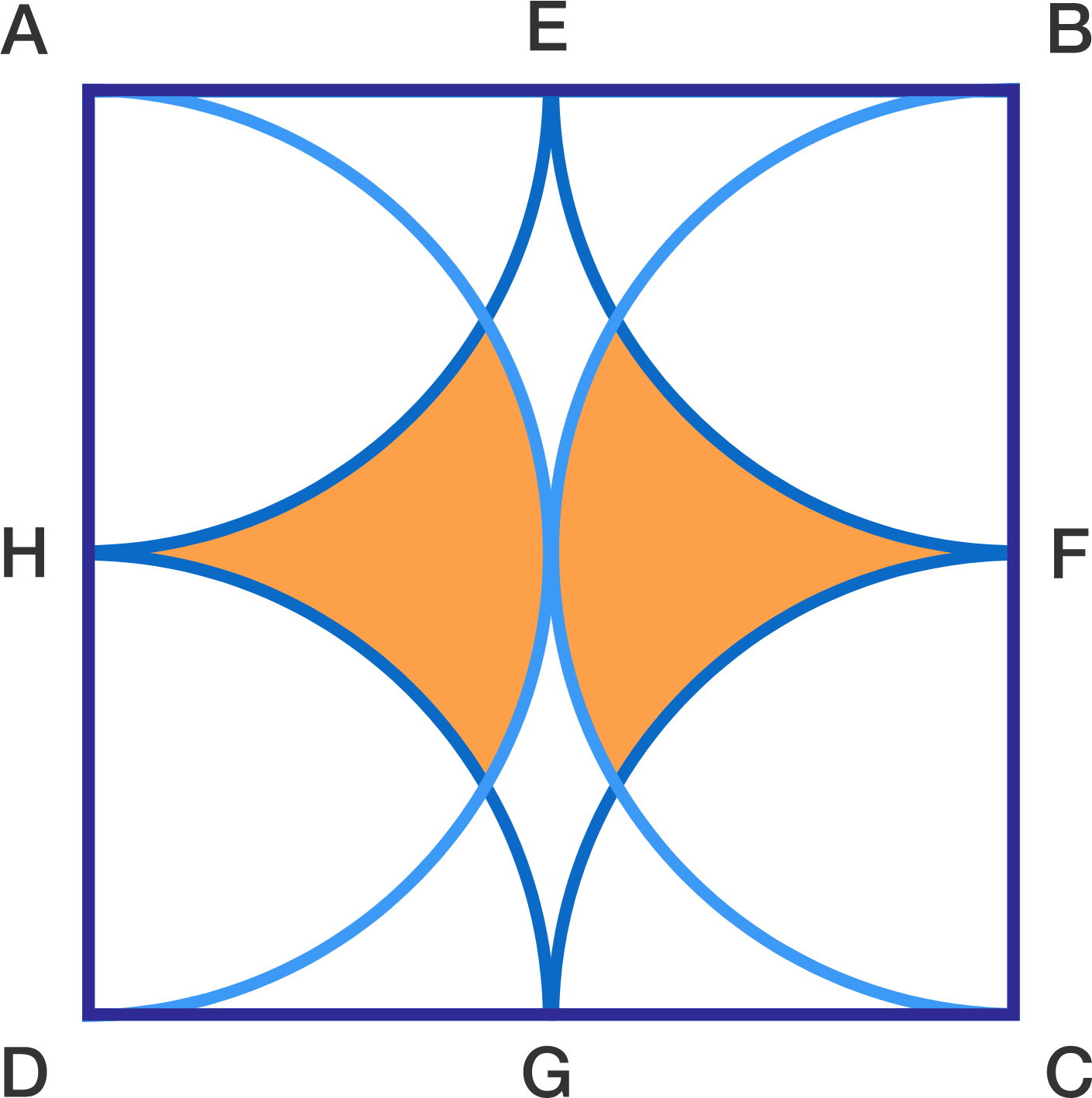# Polka Dots in Pi

Geometry Level 4In the figure, $ABCD$ is a square of side length $a$ units and $E,F,G,H$ are the midpoints of the sides.

$EAH, FBE,GCF,HDG$ are quadrants. There are two semicicles with diameters $AD$ and $BC$.

If the area of the shaded region in red can be expressed in the form

$\dfrac{a^2}x \left( \sqrt y - \dfrac\pi z\right)$

then submit $\dfrac {xy} z$ as your answer.

×

Problem Loading...

Note Loading...

Set Loading...